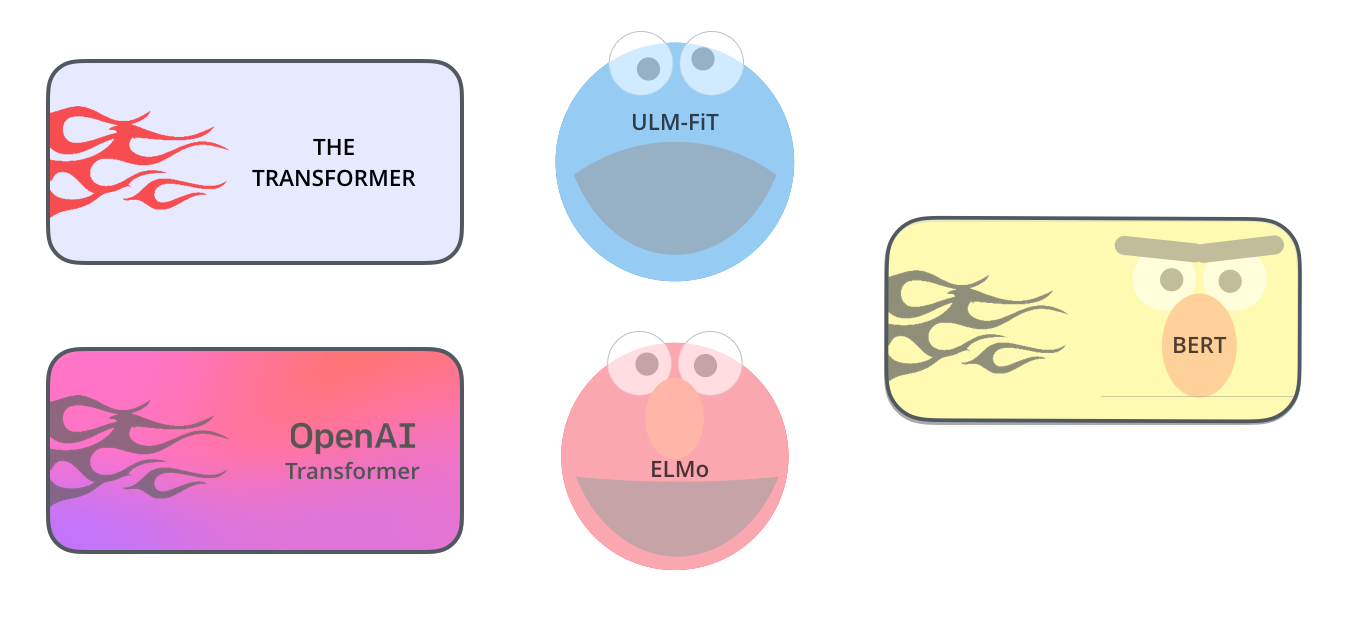## 兴奋

• 存数据
• 买（甚至开发）运算设备
• 训练模型（以天甚至月计）
• 聘用专业人员
• ……

BERT 在多项自然语言任务上测试，不少结果已经超越了人类选手。

BERT 可以辅助解决的任务，当然也包括文本分类（classification），例如情感分类等。这也是我目前研究的问题。

## 痛点

``````from sklearn.datasets import load_iris
from sklearn import tree
clf = tree.DecisionTreeClassifier()
clf = clf.fit(iris.data, iris.target)
``````

``````!git clone https://github.com/wshuyi/demo-image-classification-fastai.git
from fastai.vision import *
path = Path("demo-image-classification-fastai/imgs/")
data = ImageDataBunch.from_folder(path, test='test', size=224)
learn = cnn_learner(data, models.resnet18, metrics=accuracy)
learn.fit_one_cycle(1)
interp = ClassificationInterpretation.from_learner(learn)
interp.plot_top_losses(9, figsize=(8, 8))
``````

## 教程

Towards Data Science 专栏编辑给我私信，说：

Very interesting, I like this considering the default implementation is not very developer friendly for sure.

## 代码

Notebook 顶端，有个非常明显的 "Open in Colab" 按钮。点击它，Google Colab 就会自动开启，并且载入这个 Notebook 。

1. 你的数据，应该以 Pandas 数据框形式组织。如果你对 Pandas 不熟悉，可以参考我的这篇文章
2. 如有必要，可以调整训练参数。其实主要是训练速率(Learning Rate)和训练轮数(Epochs)。
3. 执行 Notebook 的代码，获取结果。

``````!wget https://github.com/wshuyi/demo-chinese-text-binary-classification-with-bert/raw/master/dianping_train_test.pickle

with open("dianping_train_test.pickle", 'rb') as f:
``````

``````train = train.sample(len(train))
``````

``````train.head()
``````

``````myparam = {
"DATA_COLUMN": "comment",
"LABEL_COLUMN": "sentiment",
"LEARNING_RATE": 2e-5,
"NUM_TRAIN_EPOCHS":3,
}
``````

``````result, estimator = run_on_dfs(train, test, **myparam)
``````

``````pretty_print(result)
``````

BERT 性能之强悍，可见一斑。